# 一、队列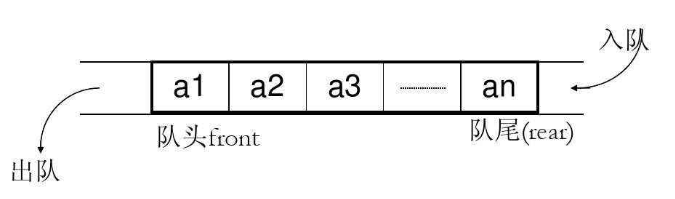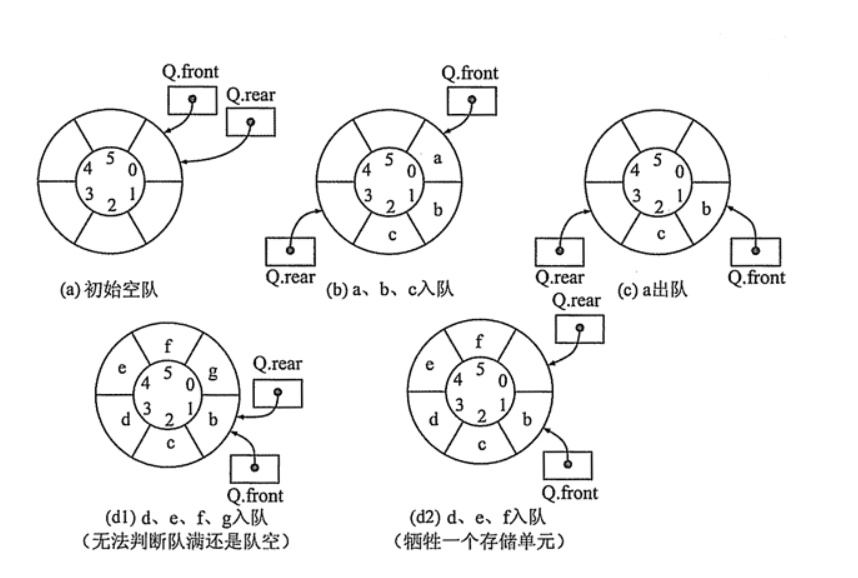• front == rear时，队为空，如图（a）
• (rear + 1) % maxsize == 0，队满。对于图（d1）的情况front == rear，无法判断到底是队空还是队满，因此需要牺牲一个存储单元，如图（d2）。
• 出队时队头指针前进1，front = (front + 1) % maxsize
• 入队时队尾指针前进1，tear = (tear + 1) % maxsize

# 二、栈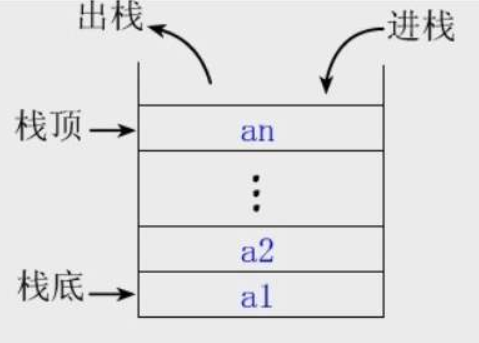• 入栈：stack.append()
• 出栈：stack.pop()
• 取栈顶：stack[-1]

# 三、例题

### 1、有效的括号

class Solution:
def isValid(self, s: str) -> bool:
map = {')':'(', ']':'[', '}':'{'}
stack = []
for char in s:
# 如果是右括号
if char in map:
top = stack.pop() if stack else '#'
if map[char] != top:
return False
# 如果是左括号
else:
stack.append(char)
return not stack

### 2、用队列实现栈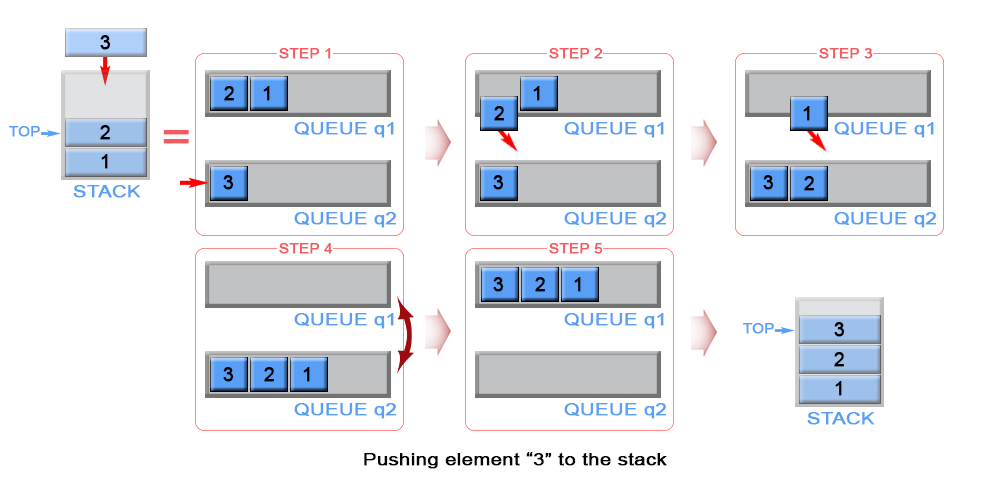from collections import deque
class MyStack:

def __init__(self):
"""
"""
self.q1 = deque()
self.q2 = deque()

def push(self, x: int) -> None:
"""
Push element x onto stack.
"""
self.q2.append(x)
while self.q1:
self.q2.append(self.q1.popleft())
self.q1, self.q2 = self.q2, self.q1

def pop(self) -> int:
"""
Removes the element on top of the stack and returns that element.
"""
return self.q1.popleft()

def top(self) -> int:
"""
Get the top element.
"""
return self.q1

def empty(self) -> bool:
"""
Returns whether the stack is empty.
"""
return len(self.q1) == 0

### 3、用栈实现队列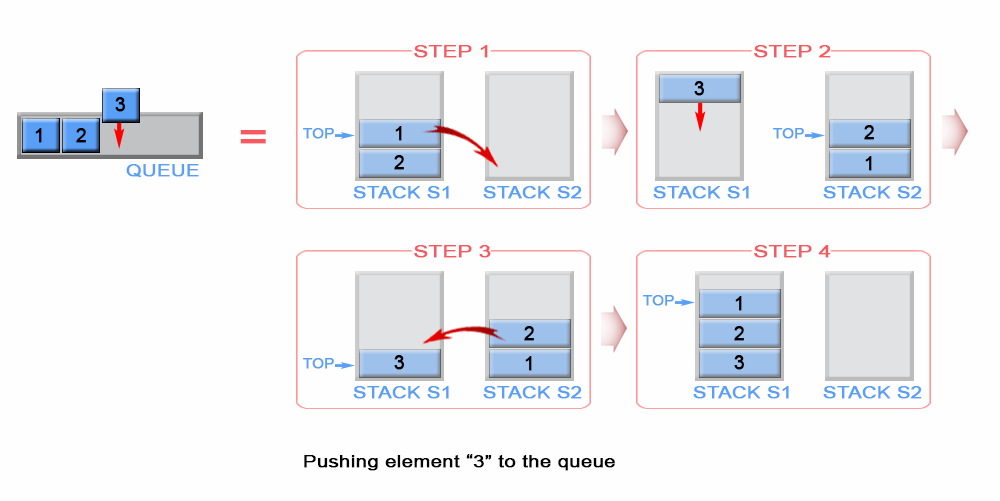class MyQueue:

def __init__(self):
"""
"""
self.s1, self.s2 = [], []

def push(self, x: int) -> None:
"""
Push element x to the back of queue.
"""
while self.s1:
self.s2.append(self.s1.pop())
self.s1.append(x)
while self.s2:
self.s1.append(self.s2.pop())

def pop(self) -> int:
"""
Removes the element from in front of queue and returns that element.
"""
return self.s1.pop()

def peek(self) -> int:
"""
Get the front element.
"""
return self.s1[-1]

def empty(self) -> bool:
"""
Returns whether the queue is empty.
"""
return len(self.s1) == 0